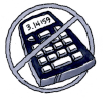### Home > MC1 > Chapter 9 > Lesson 9.1.4 > Problem9-48

9-48.

Draw angles that approximate each of the following measures.1. $50°$

This is a little more than $45°$.

1. $170°$

This is a little less than $180°$.

1. $190°$

This is a little more than $180°$.

1. $270°$

Remember that a $360°$ angle is at the center of an entire circle. Notice that $270° + 90° = 360°$.
Also, $180° + 90° = 270°$. What should this look like?